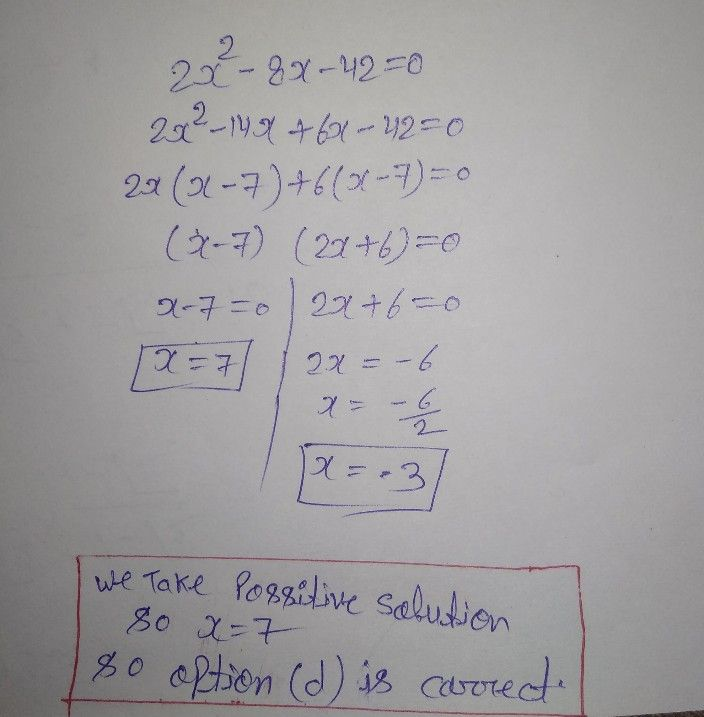Symbol
Problem$2.^{.}$ $10Q$ point What is the positive solution set of the equation $2x^{2}-8x-42=07$ $\left(-3\right)$ $\left(5\right)$ $O$ $A$ $B$ $\left(6\right)$ $\left(77y$ $=$
7th-9th grade
Other
SolutionQanda teacher - Tarunyour question is really good
ask me if you have any doubt
like my profile
dear student